# Representing a Function

There are three possible ways to represent a function:

• analytically (by formula);
• numerically (by table of values);
• visually (by graph).

Analytical representation of a function.

This is the most common way to represent a function. We define a formula that contains arithmetic operations on constant values and the variable x that we need to perform to obtain the value of y.

For example, y=1/(1+x^2), y=3x^2-5, etc.

Now, from the function y=1/(1+x^2), we can find that y(2)=1/(1+2^2)=1/5.

However, this is not the only way to represent a function. We can represent it analytically without a formula.

For example, consider the function y=[x] (floor function). Clearly, =1,[2.5]=2,[sqrt(13)]=3,[-pi]=-4, etc., but there is no formula that expresses [x].

Another example is f(x)=x! =1*2*3*...*x. The domain of this function is a set of natural numbers because x can take only natural values. So, f(3)=3! =6, but again, there is no formula that expresses x!.

Now, let's talk about the domain of the function.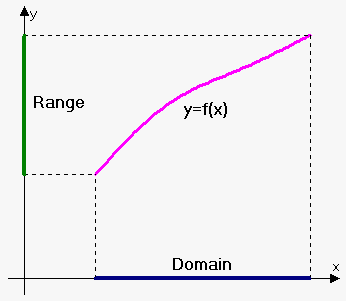We can state the domain of the function explicitly, for example, f(x)=x^2,3<=x<=5. This means that x can change only in te interval [3,5].

But often, the domain is given implicitly, i.e. the domain is all values of x where f(x) is defined.

For example, for the function f(x)=x^2+1, the domain is a set of all real numbers, i.e. the interval (-oo,oo), because the function is defined for any value of x.

The domain of the function h(x)=1/(x-2) is all real numbers except x=2, i.e. (-oo,2)uu(2,oo). x=2 is not in the domain, because h(2) is not defined (the denominator equals 0).

The domain of the function y(x)=sqrt(1-x^2) is [-1,1], because for these values the expression under the root is non-negative, and, thus, the function y(x) is defined.

Moreover, in a real-world application, we also need to make sure that the function makes sense. For example, consider the law of free fall s=1/2 g t^2. This function is defined for all t but it doesn't make sense when t<0 (time can't be negative). Therefore, the domain of the function in the given situation is (0,oo).

Example 1 . Suppose that the perimeter of the rectangle is 24 cm. Find its area as the function of one side. Find the domain of this function.

Let's suppose that one side is x and the second side is u. Then, 2x+2u=24, or u=12-x.

Therefore, the area is A=xu=x(12-x). Thus, A(x)=x(12-x).

Since the length should be positive, x>0. Also, the area should be positive; so, 12-x>0, or x<12. Thus, the domain is the interval (0,12).

Let's work another quick example.

Example 2 . State the domain of f(x)=1/(x^2-3x).

Since x^2-3x=x(x-3) and the denominator can't equal 0, the domain of the function is all real numbers except x=0 and x=3.

One more quick example for practice.

Example 3. Find the domain of the function f(t)=sqrt(t)+root(3)(t).

Since the square root should be non-negative, the domain of the function is t>=0.

And let's do another short example before passing on.

Example 4. Find f(x+h) if f(x)=sqrt(x)/(x^2+3x+4).

f(x+h)=sqrt(x+h)/((x+h)^2+3(x+h)+4).

Numerical (tabular) representation of a function.

In real life, the dependence between variables is formed based on experiments or observations. For example, at any given time t, we can calculate the world population N. We say that N is a function of t but can't find an explicit formula to express this. All we have is a table of values as below:

 t (in years) 1970 1980 1990 2000 2010 N (in millions of people) 3835 4100 4545 5600 7300

However, in further topics of calculus, we will be able to find a function that will approximate these values.

Graphical representation of a function.

Although in calculus functions are not defined graphically, graphical representation is very helpful in studying functions.

Suppose that we are given a function y=f(x) with the domain X. Imagine a plane with two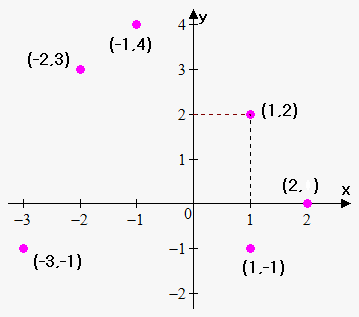perpendicular axes: the x-axis and the y-axis. Consider a pair of corresponding values x and y, where x is taken from set X and y=f(x). The image of this pair is the point A(x,y) with the x-coordinate x and the y-coordinate y. When the variable x is changing within the interval X, this point describes some curve. This curve is the graph of the function y=f(x).

So, to draw the graph of the function, take from the interval X points that are close to each other: x_1,x_2,x_3,...x_n. Now, find the corresponding y-values: y_1=f(x_1),y_2=f(x_2),...,y_n=f(x_n).

Draw the points (x_1,y_1),(x_2,y_2),...(x_n,y_n). Connect these points by a smooth curve. We've obtained the graph of the function. Note: the more points you take, the more accurate graph you will obtain.

Let's move on to practical examples.

Example 5. Draw the graph of the function y=x^2 on the interval [0,1].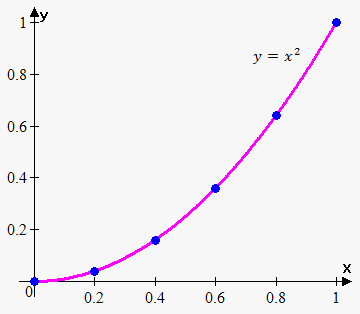Let's take the following points: x_1=0,x_2=0.2,x_3=0.4,x_4=0.6,x_5=0.8,x_6=1.

Now, find the corresponding y-values: y_1=(0)^2=0, y_2=(0.2)^2=0.04, y_3=(0.4)^2=0.16, y_4=(0.6)^2=0.36, y_5=(0.8)^2=0.64, y_6=(1)^2=1.

Draw the points (0,0),(0.2,0.04),(0.4,0.16),(0.6,0.36),(0.8,0.64),(1,1) on the xy-plane and connect them by a smooth curve.

And a bit more work to memorize it better.

Example 6. Consider the function whose graph is shown to the left. Find f(0), f(2) and state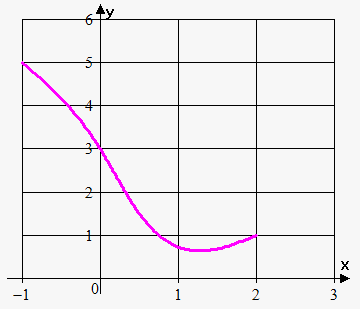the domain and the range of f.

We take the point x=0 and move up until the intersection of the curve. The intersection is at the point 3; so, f(0)=3. Similarly, f(2)=1.

The function is defined when -1<=x<=3; therefore, the domain of the function is the interval [-1,3], and the range is [1,5.5].

The graph of the function is a curve in the xy-plane. But the question arises: which curves in the xy-plane are graphs of functions? This is answered by the following test.

Vertical line test. A curve in the xy-plane is the graph of a function of x if and only if no vertical line intersects the curve more than once.

Graphical representation allows us easily to determine whether the curve is a function.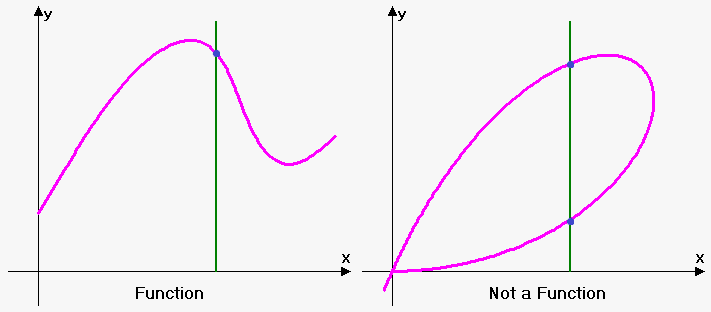Consider the graph of the function y^2+x^2=4. It is a cirlcle with a radius of 2. Clearly, it is not a function.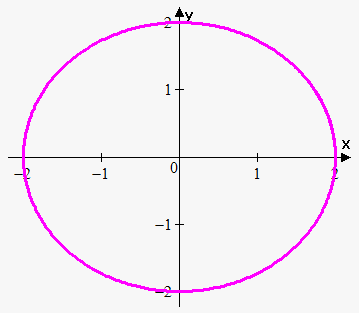To see why it is so, express y in terms of x: y^2=4-x^2, or y=+-sqrt(4-x^2). Because of +-, we have two values of y for every value of x. Also, as can be seen from the graph, the function fails the vertical line test.

Parametric representation of a function.

Parametric representation is a slightly changed form of representing a function by a formula.

When we define a function by a formula, we use the following record: y=f(x), where f is some formula.

But often, it is more convenient to represent a function using two functions and a parameter.

If we define a function as x=u(t),y=v(t), by changing the value of t (parameter), we can find the corresponding pairs of x and y.

In general, we set some restrictions on t. If we require a<=t<=b, the point (u(a),v(a)) is called initial, and the point (u(b),v(b)) is called terminal.

In some cases (but not always), it is possible to eliminate the paremeter t and obtain the standard representation of y as a function of x (or x as a function of y).

Also, note that parametric equations can generate curves that are not functions.

Example 7. Draw graph of the function x=t^3+1,y=t^2-1, -2<=t<=2.

Let's find a couple of points that correspond to different values of t.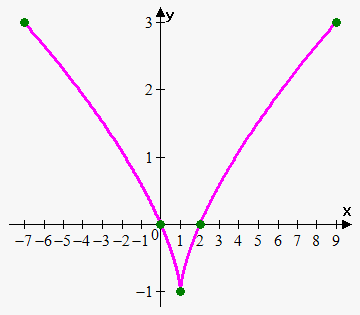t x y -2 (-2)^3+1=-7 (-2)^2-1=3 -1 (-1)^3+1=0 (-1)^2-1=0 0 0^3+1=1 0^2-1=-1 1 1^3+1=2 1^2-1=0 2 2^3+1=9 2^2-1=3

The graph of this function is shown to the right.

Note that in this case we can eliminate the parameter t: from the first equation, t=(x-1)^(1/3); plugging this equation for t into the second equation gives y=(x-1)^(2/3)-1.

And one more final example.

Example 8. Draw the function x=t^2+t,y=t^3-t^2, -2<=t<=2.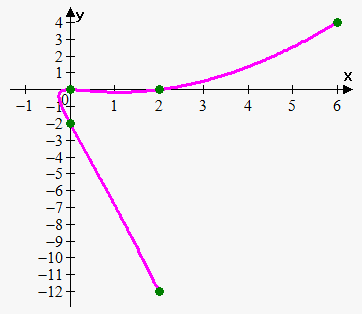Let's find a couple of points that correspond to different values of t.

 t x y -2 (-2)^2+(-2)=2 (-2)^3-(-2)^2=-12 -1 (-1)^2+(-1)=0 (-1)^3-(-1)^2=-2 0 0^2+0=0 0^3-0^2=0 1 1^2+1=2 1^3-1^2=0 2 2^2+2=6 2^3-2^2=4

Note that this is not a function, because it fails the vertical line test. Also, it is very hard to eliminate the parameter t.